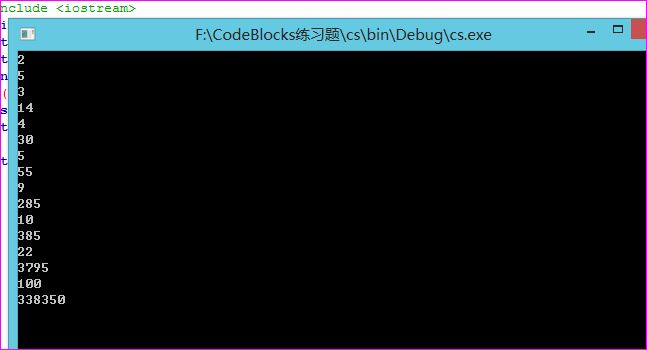函数递归之用递归方法求 f(n)

题目描述

C语言习题 用递归方法求 f(n) = 12+22+...+n2  ,n的值由主函数输入。

n的值。

输出

f(n) 的值。#include <iostream>

using namespace std;

int fac(int n)

{

int a;

if(n==1) a=1;

else a=fac(n-1)+n*n;

return(a);

}

int main()

{

int n;

cin>>n;

cout<<fac(n)<<endl;

return 0;

}